#Quality Digest

Featured Product
This Week in Quality Digest Live
Statistics Features
Donald J. Wheeler
What do predictable processes have in common with chaos?
William A. Levinson
People can draw the wrong conclusions due to survivor, survey, and bad news bias.
Donald J. Wheeler
Does your approach do what you need?
Paul Laughlin
Correlation vs. causality
Donald J. Wheeler
In spite of what everyone says to the contrary
Statistics News
New capability delivers deeper productivity insights to help manufacturers meet labor challenges
Day and a half workshop to learn, retain, and transfer GD&T knowledge across an organization
Elsmar Cove is a leading forum for quality and standards compliance
InfinityQS’ quality solutions have helped cold food and beverage manufacturers around the world optimize quality and safety
User friendly graphical user interface makes the R-based statistical engine easily accessible to anyone
Collect measurements, visual defect information, simple Go/No-Go situations from any online device
Good quality is adding an average of 11 percent to organizations’ revenue growth
Ability to subscribe with single-user minimum, floating license, and no long-term commitment
A guide for practitioners and managersBio

Statistics

## Some Outlier Tests, Part 1

### Comparisons and recommendations

Published: Monday, December 7, 2020 - 13:03

All articles in this series:

The first statistical test was a test for outliers. The problem of what to do about outliers has been around from the beginnings of data analysis. Part one will compare four tests for outliers. Next month part two will cover some additional tests for outliers.

Statisticians know how to analyze homogeneous data. After all, the initial assumption behind all statistical inference is that the data are represented by independent and identically distributed random variables. But all outliers are prima facie evidence of a lack of homogeneity. As such, outliers undermine statistical analyses. Hence statisticians often seek to sharpen up their analyses by deleting the outliers.

But what if the outliers are more than some sort of clerical error? What if the outliers are actually good data that reflect a change in the process or system producing the measurements? The purpose of analysis is insight, but what insight can be gained if we ignore signals of a change? So while the detection of outliers is important, the assumption that we can delete the outliers and then obtain a meaningful analysis is highly questionable. Perhaps the greatest insight to be obtained from our data is an explanation of why the outliers exist. Good experimenters understand this. To paraphrase George Box, if you discover silver while digging for gold, stop and mine the silver. Nevertheless, whether we delete the outliers and proceed with our statistical computations, or stop to learn why the outliers happened, the first step is still the detection of the outliers.

### Peirce’s criterion

In 1852, Benjamin Peirce published a test for identifying outliers1. His test was equivalent to the following: Given n data (for n = 4 to 60) compute the average and the global standard deviation statistic. Let k denote the number of suspected outliers (k = 1 to 9) and compute the interval:

Average ± R(n,k)Standard Deviation Statistic

where the R(n,k) values can be found in a table in reference2. Regardless of the value of k used, any and all values found outside the interval above are classified as outliers. Figure 1 contains a few of the R(n,k) values. Inspection of figure 1 reveals that Peirce's criterion defines outliers as points that fall as little as 1.2 to 2.4 standard deviations away from the average. Figure 2 contains the estimated overall alpha levels for these R(n,k) values.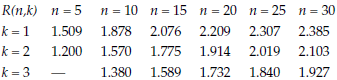Figure 1: Some R(n,k) factors for Peirce’s criterion for outliersFigure 2: Overall alpha levels for Peirce’s criterion for outliers

The overall alpha levels in the first row are unacceptably high by modern standards and the remaining alpha levels are, in a word, obscene. When we perform a test with a false alarm probability of 35 percent, 70 percent, or 90 percent we completely undermine the likelihood that an observed outcome is real. This likelihood that a positive test outcome is correct is known as the positive predictive value (PPV) for the test. In this context the PPV is the probability that a point identified as an outlier is actually an outlier rather than a good data value. The PPV curve for Peirce’s R(n,1) values is shown in figure 3. (See the postscript for more about the PPV curves.)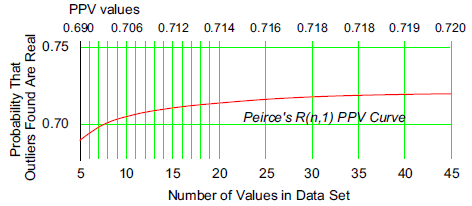Figure 3: PPV curve for Peirce’s R(n,1) cutoffs

When we use Peirce’s test and identify a point as an potential outlier, we have no more than a 72-percent chance that that point really is an outlier. There is at least a 28-percent chance that it is actually a good data value that has been misidentified. The second and third rows of figure 1 have PPV curves that are much lower than the curve shown in figure 3. About the only thing worse than using Peirce’s test for a single outlier (where k = 1) is using Peirce’s test with k > 1.

You have paid good money to obtain your data, and you will want to be sure that a point is an outlier before you delete it, but Peirce’s large alpha levels will always deny you this assurance. Moreover, there is a differential priority for finding outliers. As we saw last month the contaminating effect of an outlier increases with the size of the outlier3. Specifically, if we add a single outlier to a data set of n values we get: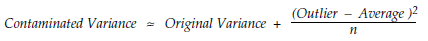This equation shows why it is more important to detect the larger outliers. In spite of this, Peirce’s test is so focused on finding all of the outliers that it virtually guarantees that you will also have false alarms at least 36 percent of the time. As we will see below, better tests for outliers exist. Yet Peirce’s criterion is still being recommended in articles published within the last 20 years2.

### Chauvenet’s criterion

In 1863, William Chauvenet published another test for identifying outliers4. His test was equivalent to the following: Given n data (n ≥ 5) compute the average and the global standard deviation statistic. Let z(n) denote the standard normal variate that corresponds to the upper tail probability P = [1/(4n)]. (For n = 5, P = 0.05, and z(n) = 1.645.) Then compute the interval:

Average ± z(n) Standard Deviation Statistic

Values outside this interval are designated as outliers. Figure 4 gives selected values of z(n), the estimated overall alpha levels, and the PPV values.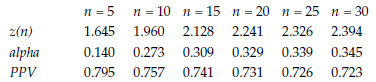Figure 4: Chauvenet’s criterion for outliers

Chauvenet’s criterion may be seen to be slightly more conservative than Peirce’s by comparing the z(n) values with the R(n,1) values. However, Chauvenet’s overall alpha levels quickly reach the neighborhood of 30 percent, resulting in the excessive identification of good data as outliers. The PPV curve for Chauvenet’s test is shown in figure 5. It drops down with increasing n because Chauvenet’s test does not try to hold the overall alpha level constant.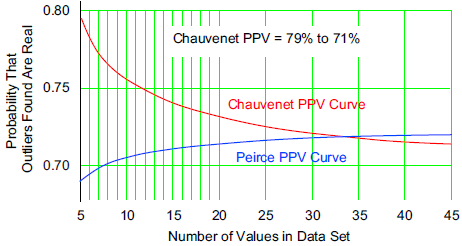Figure 5: PPV curve for Chauvenet’s criterion for outliers

Overall, Chauvenet’s test leaves you with about a 71 percent to 79 percent chance that the values you identify as outliers may actually be real outliers. While this is slightly better than Peirce’s PPV curve, it is still not very good.

In all fairness, at the time Peirce and Chauvenet did their work, the global root-mean-square deviation was all that was available. The work that resulted in the use of the within-subgroup variation began in 1875 with Sir Frances Galton’s sweet-pea experiment but was not fully developed until the early twentieth century. So Peirce and Chauvenet did not know about the modern foundation of statistical inference when they developed their techniques. Today we do, and so we can do better. Nevertheless, Chauvenet’s testcontinues to be included in articles and textbooks today2,5,6.

### The interquartile range test for outliers

In 1977, John W. Tukey gave us a nonparametric test for “outside” values with fixed-width limits based on the Interquartile Range7. This test begins with the data arranged in numerical order and uses the first and third quartiles. The interquartile range is the difference between the third quartile and the first quartile:

IQR = Q3 – Q1

Tukey’s upper cutoff point is Q3 + 1.5 IQR.

Tukey’s lower cutoff point is Q1 – 1.5 IQR.

Points outside these cutoffs are classified as outliers. For a normal probability model these cutoffs approximate the interval:

Average ± 2.700 Standard Deviations

While ±2.7 standard deviations sounds more conservative than either Peirce’s or Chauvenet’s tests, the inherent uncertainty in the quartile statistics results in a technique that is comparable in performance.

Since the first and third quartiles will depend upon the next-to-largest and next-to-smallest values when n = 5, 6, 7, or 8, this test can only detect extremely large outliers when it is used with small data sets.

The IQR test does not attempt to control the overall risk of a false alarm. Figure 6 lists some estimated alpha-levels and PPV values for this procedure. Once more we are faced with alpha-levels in the neighborhood of 30 percent or more which will inevitably result in many false alarms.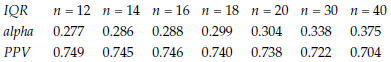Figure 6: False alarm rates for the interquartile range test for outliers

The PPV curve for the IQR test is shown in figure 7. It falls in the same neighborhood as Peirce’s and Chauvenet’s tests. While John Tukey only proposed this as a preliminary technique, its large overall alpha levels and weak PPV makes this test unsatisfactory for any serious analysis.Figure 7: PPV curve for IQR test for outliers

The fact that such weak procedures as Peirce’s and Chauvenet’s procedures are still getting mentioned in engineering texts and journal articles is a testimony to the need to be able to detect unusual values. While unusual values appear in all sorts of data, this is no excuse for using inferior techniques for detecting them. Better techniques are available. One of these is given below, and others will be given in part two.

### The XmR chart test for outliers

The chart for individual values and moving ranges was created by W. J. Jennett in 1942 as a sequential procedure for tracking a continuing stream of individual values8. However, the baseline portion, where the limits are computed, may be used as a stand-alone test for outliers with data sets of 8 or more values9. Here we use fixed-width limits defined by:

Average  ±  3  *  ( Average Moving Range / 1.128)

Points outside these limits may be safely interpreted as being outliers.

This approach differs from all of the preceding approaches in that it is built on the foundation of all modern techniques of statistical inference: it uses the within-subgroup variation rather than a global measure of dispersion. By using John von Neumann’s method of successive differences it avoids the contamination that is inherent with all global measures of dispersion10.

When we use the baseline portion of an XmR chart as a test for outliers the overall alpha level will increase with increasing n as shown in figure 8. However, this overall alpha level does not grow as large or as rapidly as the overall alpha levels for Peirce’s, Chauvenet’s, and the IQR tests. Thus, when the XmR chart identifies a point as an outlier it is very likely to actually be an outlier. The PPV values for the XmR chart are shown in figure 8 and plotted in figure 9.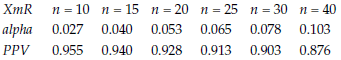Figure 8: Characteristics of the XmR test for outliers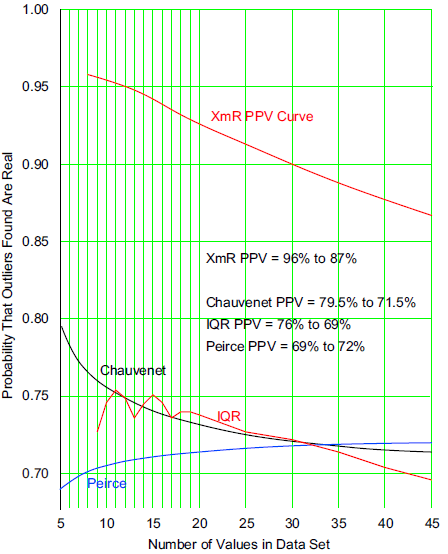Figure 9: PPV curve for XmR test for outliers

If you use the baseline portion of an XmR chart as a test for outliers you will find those outliers that are large enough to create serious contamination while simultaneously minimizing the number of times that good data are misidentified as outliers. The result is a PPV curve that is much higher than the curves for the preceding tests. As a test for outliers, the baseline portion of an XmR chart is superior to all of the preceding tests. When you find an outlier it is very likely to be real.

With data sets having n = 5, 6, or 7 values which contain a single value that looks different from the rest, the XmR chart limits may be computed using the n–1 values and the different value may be compared to these limits. The conservative nature of the XmR limits when used with short baselines is sufficient to label a point outside these jackknifed limits as an outlier.

Caution: Since the method of successive differences does not work when the data have been arranged in numerical order, it is important to avoid applying an XmR test for outliers to data sets where the values have been arranged in ascending or descending order. The natural time order for the data is generally used with the XmR test. However, if the natural time order is unknown, any arbitrary ordering may be used as long as it does not depend upon the values themselves.

So, the baseline portion of an XmR chart is not only easier to use than Peirce’s test, Chauvenet’s test, or the IQR test, but it also outperforms them. The XmR chart simply does a better job of striking a balance between the twin errors of missing a signal and getting a false alarm. The baseline portion of an XmR chart provides a test for outliers that allows you to have confidence in the results. If you want simplicity with superior performance, the XmR chart may be all you need.

### Impossible tests

Peirce’s test and Chauvenet’s test use the global standard deviation statistic. As we learn from Shiffler11 the computation of this statistic imposes an upper bound on the size of a standardized observation from a set of n data: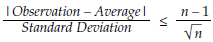For n = 3 this maximum value is 1.1547. This means that when n = 3 no data point can ever fall outside the interval:

Average ± 1.1547 Standard Deviations

Peirce’s criterion has a cutoff for n = 3 of R(3,1) = 1.196. Since this value exceeds the maximum value of 1.1547, Peirce’s test for n = 3 will never find an outlier! Peirce’s criterion simply does not work for n = 3.

Chauvenet’s criterion has a cutoff for n = 3 of z(3) = 1.383 which also exceeds the maximum of 1.1547. In addition, Chauvenet’s cutoff for n = 4 of z(4) = 1.534 exceeds the maximum value for n = 4 of 1.500. Thus, Chauvenet’s test can never detect an outlier when n = 3 or n = 4! Chauvenet’s criterion simply does not work for either n = 3 or n = 4.

So, while you may find tables that list critical values for these tests for n = 3 and n = 4, these critical values are purely theoretical values that cannot ever be attained in practice.

In addition, there is the problem of chunky data to be considered. Before Peirce’s test will work as advertised with n = 4 the standard deviation statistic will need to be greater than 17 times the measurement increment present in the data. Before Chauvenet’s test will work as advertised with n = 5 the standard deviation statistic will need to be greater than 14 times the measurement increment present in the data. And in order for the XmR test to work as intended the average moving range will need to be larger than 0.9 times the measurement increment present in the data. These restrictions will be explained in part two.

### Summary

Peirce’s criterion is so focused on finding outliers that, when k > 1, everything begins to look like an outlier. And when k = 1 the excessive overall alpha levels undermine the likelihood that a point identified as an outlier is actually an outlier. If you are looking for an explanation for the outliers this wastes time and effort, and if you are deleting the outliers this results in the deletion of good data. Anyone who seriously suggests using Peirce’s criterion must be classified as statistically naive. Peirce’s criterion is an inferior technique with serious problems in practice.

Chauvenet’s criterion is only slightly more conservative than Peirce’s criterion for k = 1. It suffers from the same problems. It does a very poor job of separating the interesting outliers from the non-outliers. Again, while this test is being used today, it should not be. It has nothing to recommend it except the window dressing of apparent mathematical rigor.

The IQR test avoids the tables of scaling constants by using fixed-width limits. However this simplicity is offset by the inherent uncertainty in the quartile statistics. The result being a test that in practice performs very much like both Peirce’s test and Chauvenet’s test.

Using the baseline portion of an XmR chart offers the simplicity of fixed-width limits combined with efficient measures of location and dispersion. The within-subgroup variation is more robust to the presence of outliers than the global standard deviation, resulting in a better separation between the potential outliers and the routine variation. With smaller overall alpha levels, and with better PPV values, this test outperforms the other tests given here by a wide margin.

Part two will consider some tests for outliers that hold the overall alpha level constant.

### Why we use the normal distribution

When developing an outlier test we use the normal distribution as our model for a data set with no outliers. We do this because the normal distribution is the distribution with maximum entropy. This means that the outer 10 percent of a normal distribution is further away from the average than the outer 10 percent of any other probability model12,13. So if we have a test that will effectively separate the outer 10 percent of a normal distribution from outliers, then we will have a test that can be expected to work reasonably well with virtually every other probability model where the central 90 percent of the data are more closely clustered about the average.

### Postscript on finding PPV values

The PPV curves were computed using the neutral a priori assumption of a 50-percent likelihood for an outlier. The power curves for each test criterion for n = 5 (1) 20 (5) 45 were combined with the a priori values to compute PPV values using outliers ranging in size from 3 sigma to 6 sigma. Next these specific PPV values were averaged for each n to obtain the PPV values used to produce the curves.

References

1. Benjamin Peirce, “Criterion for the Rejection of Doubtful Observations,”
Astronomical Journal II, v.45, pp. 161–163, 1852.

2. Stephen M. Ross, “Peirce’s Criterion for the Elimination of Suspect Experimental Data,” Journal of Engineering Technology, Fall 2003.

3. Donald J. Wheeler, “The Global Standard Deviation Statistic,”
Quality Digest, November 2, 2020.

4. William Chauvenet, A Manual of Spherical and Practical Astronomy Vol. II,
Lippincott, Philadelphia 1863: Reprint of 1891 Fifth Ed., Dover, N.Y. 1960.

5. John Taylor, Error Analysis, 2nd Ed., pp. 166-170,
University Science Books, Sausalito, California, 1997.

6. J.P. Holman, Experimental Methods for Engineers, 7th Ed., pp. 78-80, McGraw Hill, 2001.

7. John W. Tukey, Exploratory Data Analysis, pp. 43–44,

8. J. Keen, D. Page, “Estimating Variability from the Differences Between Successive Readings,Applied Statistics, v.2, 1953, pp. 13–23.

9. William H. Woodall, “A Note on Maximum z-Scores for Control Charts for Individuals,” Communications in Statistics—Theory and Method, v. 21. pp. 3211–3217, 1992.

10. J. von Neumann, et. al., “The Mean Square Successive Difference,”
Annals of Mathematical Statistics, v.12, 1941, pp. 153–162.

11. R.E. Shiffler, “Maximum z-Scores and Outliers,”
American Statistician, v. 42, pp. 79–80, 1988.

12. Donald J. Wheeler, “What They Forgot to Tell You About the Normal Distribution,”
Quality Digest Daily, September 6, 2012.

13. Donald J. Wheeler, “The Heavy-Tailed Normal,” Quality Digest Daily, October 1, 2012.

### Discuss